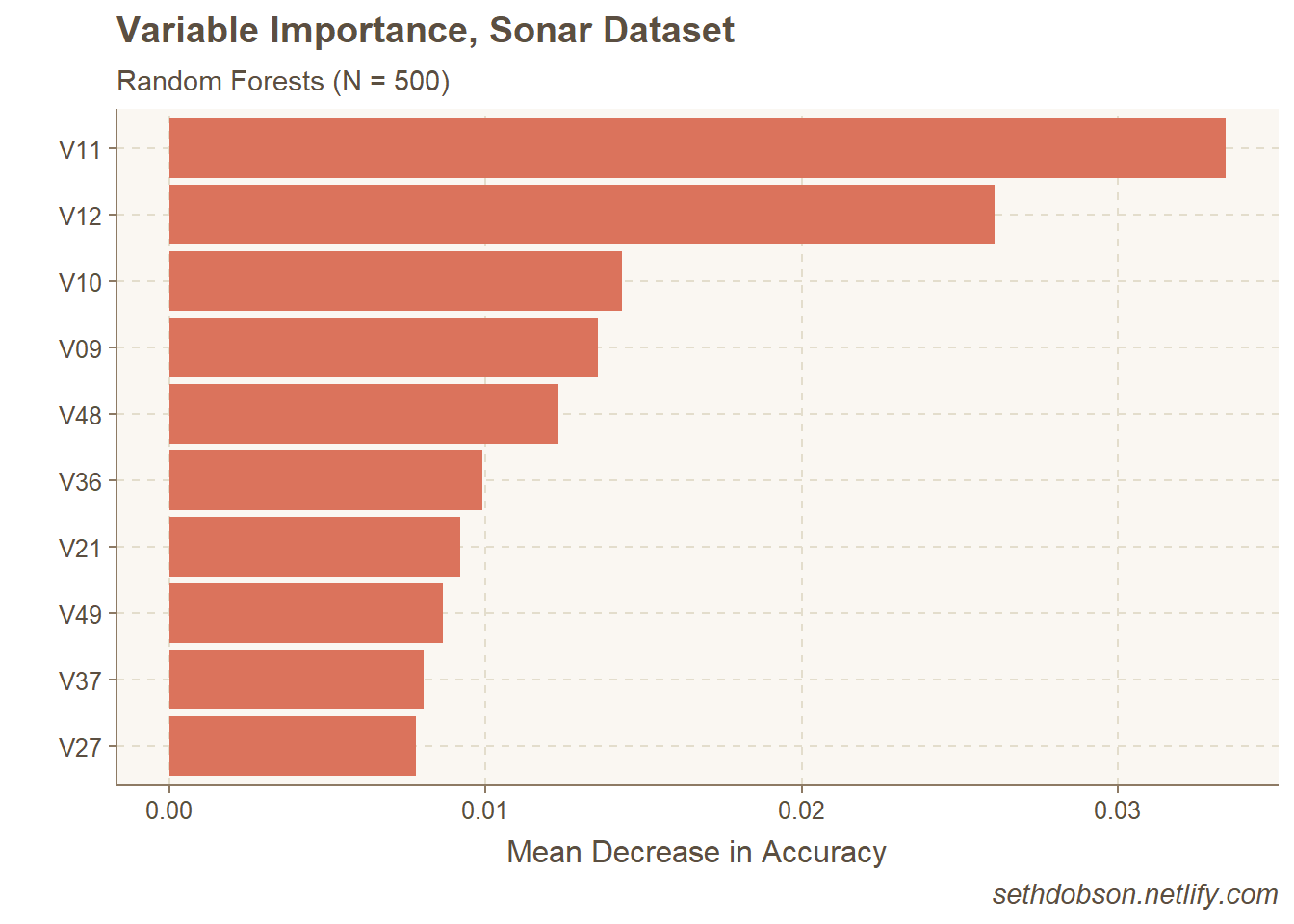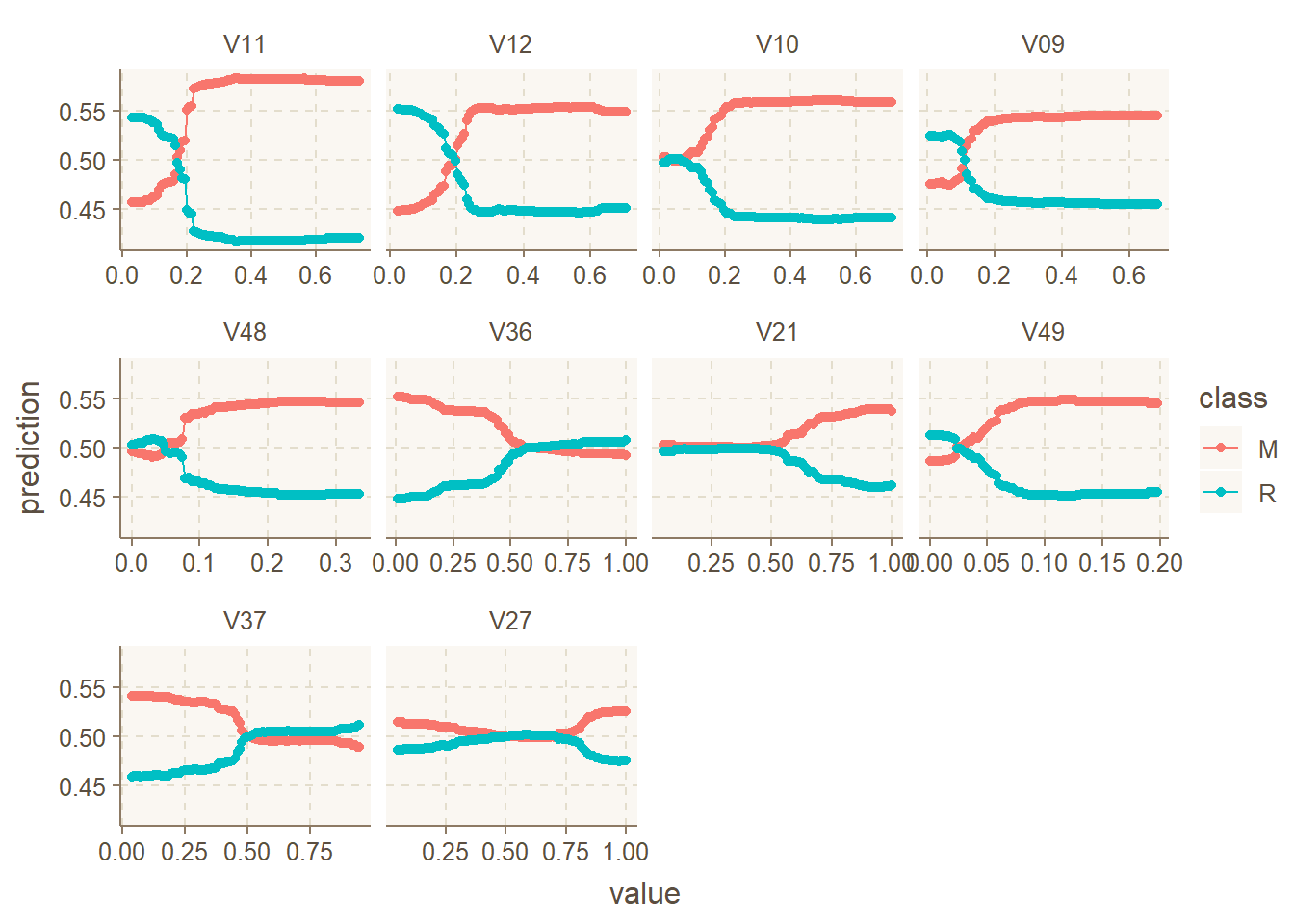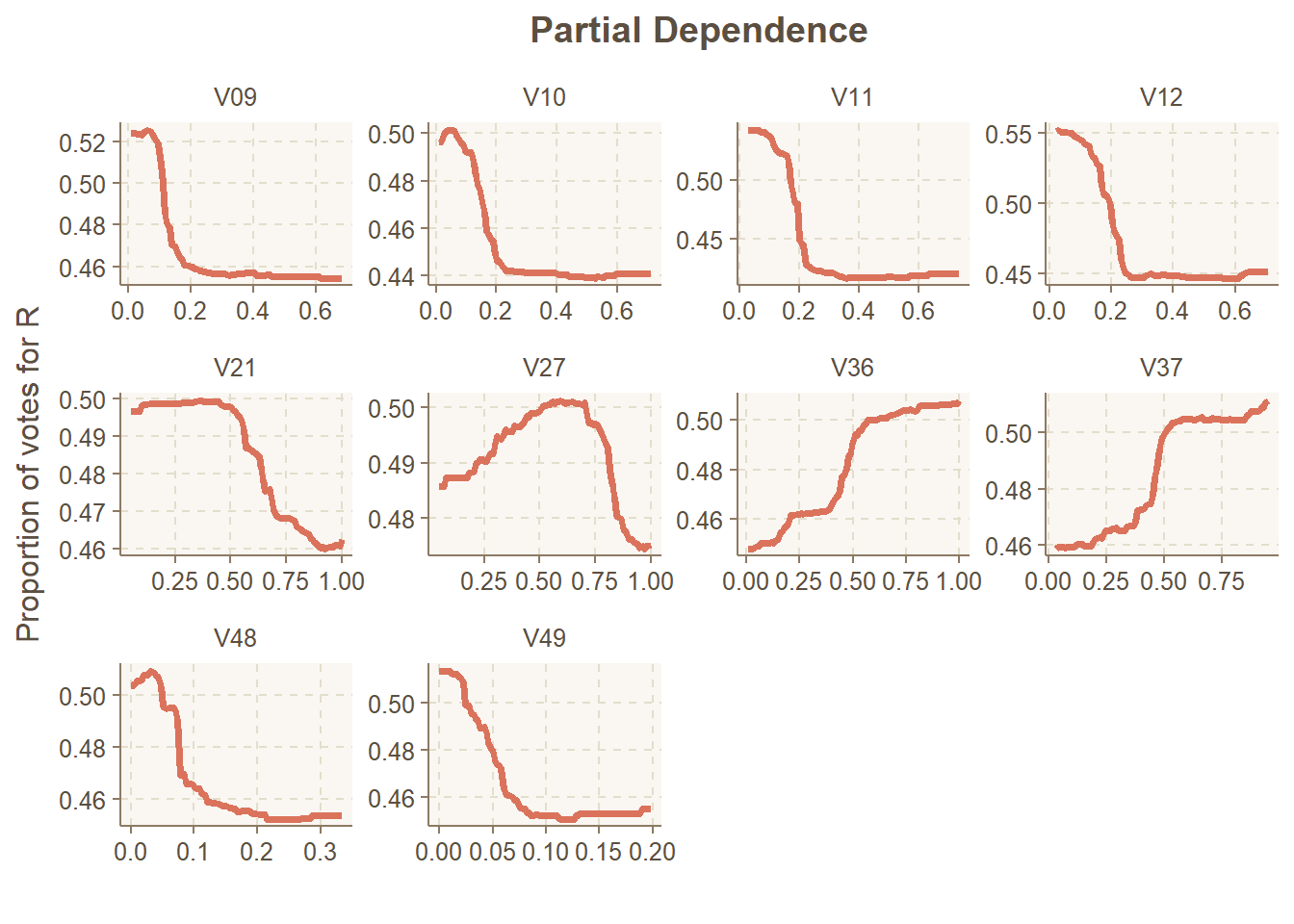# In search of the perfect partial plot

## Introduction

Partial dependence (PD) plots are essential for interpreting Random Forests models. For example, in the case of binary classification, PD plots show the marginal effect of individual predictor variables on the probability of the response.

Several packages in R will generate PD plots for Random Forests, but I’ve never been completely satisfied with any of them, until now.

In this post, I show how I created a customized PD plot function with the help of ggplot2 and the edarf package, thus ending my long search for the perfect Random Forests PD plot in R.

## Why I love edarf

My biggest gripe with most PD plot functions in R is how slow they are. In my job, I am usually working with more than 100,000 rows at a time building predictive models. So, not Big Data, but big enough that running computationally intensive functions on my local drive can take a while.

For example, the partialPlot() function in the randomForest package can take an hour or more to produce PD plots for several predictors when N > 100,000. In contrast, edarf::partial_dependence() does the same job in a matter of seconds!

The partial_dependence() function in edarf uses the marginalPrediction() function from the mmpf package to calculate the marginalized effect of a given predictor on the Random Forests “vote” proportion using Monte Carlo integration. I assume that the use of a Monte Carlo method is what makes edarf::partial_dependence() so fast. But I’m not a mathematician, so don’t hold me to that.

## Just one problem

My only beef with edarf is that I don’t love the plots. Let’s take a look at an example to show you what I mean.

I am going to train a Random Forests binary classifier using the Sonar dataset from the mlbench package. The dataset contains 60 numeric predictor variables representing different sonar signals bounced off either a metal cylinder or a roughly cylindrical rock. The response variable has two classes: M for metal cylinder or R for rock.

# Load packages and set theme
library(tidyverse)
library(mlbench)
library(randomForest)
library(caret)
library(edarf)
library(rlang)
library(ggthemr)
ggthemr("dust")

data("Sonar")
df<-Sonar
rm(Sonar)

# Clean up variable names (becuz I'm a bit OCD)
df <- df %>% rename(V01 = V1, V02 = V2, V03 = V3, V04 = V4,
V05 = V5, V06 = V6, V07 = V7, V08 = V8,
V09 = V9)

I will use the randomForest() function from the randomForest package to build the classifier.

# Get minimum class frequency
min <- min(table(df$Class)) set.seed(223) df_rf <- df %>% na.omit() fit_rf <- randomForest(data = df_rf, Class ~ ., ntree = 500, importance = TRUE, sampsize = c(min, min)) Notice that I have downsampled the most frequent class, as I have found that this usually gives higher balanced accuracy compared to resampling based on the observed class probabilities. Let’s take a look at the out-of-bag (OOB) performance using caret::confusionMatrix(). # Add predicted values to data frame df_rf <- df_rf %>% mutate(predicted = predict(fit_rf)) # Get performance measures confusionMatrix(df_rf$predicted, df_rf$Class, positive = "R") ## Confusion Matrix and Statistics ## ## Reference ## Prediction M R ## M 100 17 ## R 11 80 ## ## Accuracy : 0.8654 ## 95% CI : (0.8114, 0.9086) ## No Information Rate : 0.5337 ## P-Value [Acc > NIR] : <2e-16 ## ## Kappa : 0.7285 ## ## Mcnemar's Test P-Value : 0.3447 ## ## Sensitivity : 0.8247 ## Specificity : 0.9009 ## Pos Pred Value : 0.8791 ## Neg Pred Value : 0.8547 ## Prevalence : 0.4663 ## Detection Rate : 0.3846 ## Detection Prevalence : 0.4375 ## Balanced Accuracy : 0.8628 ## ## 'Positive' Class : R ##  The model has decent predictive performance. So that’s nice. I generally like to make PD plots for just the top predictors in a Random Forests model. So next let’s get the variable importance of each predictor using the randomForest::importance() function, and plot the top 10 based on mean decreased accuracy (note: the values are not scaled by their standard deviations). # Get variable importance measures imp_df <- data.frame(importance(fit_rf, scale = FALSE, type = 1)) # Tidy up and sort the data frame imp_df <- imp_df %>% mutate(names = rownames(imp_df)) %>% arrange(desc(MeanDecreaseAccuracy)) # Plot mean decreased accuracy imp_df %>% top_n(10, MeanDecreaseAccuracy) %>% ggplot(aes(x = reorder(names, MeanDecreaseAccuracy),y = MeanDecreaseAccuracy)) + geom_col() + coord_flip() + labs(title = "Variable Importance, Sonar Dataset", subtitle = "Random Forests (N = 500)", x= "", y= "Mean Decrease in Accuracy", caption = "sethdobson.netlify.com") + theme(plot.caption = element_text(face = "italic"))Figure 1: Top 10 most important predictors As you can see in Figure 1, V11 and V12 stand out as being the most important predictors in the model. Now that we know which predictors are the most important, we can save the variable names as a character vector and pass it along to edarf::partial_dependence(). The resulting data frame will contain marginalized probabilities (vote proportions) at each point along a user-specified uniform grid. # Save top predictor names as character vector nm <- as.character(imp_df$names)[1:10]

# Get partial dependence values for top predictors
pd_df <- partial_dependence(fit = fit_rf,
vars = nm,
data = df_rf,
n = c(100, 200))

Note that n according to the mmpf documentation is “an integer vector of length two giving the resolution of the uniform or random grid on vars for the first element, and the number of the rows of the data to be sampled without replacement for the second element.”

I chose 100 for the first number because I like to visualize marginal effects over centiles of the predictor variables.

Now we can use the edarf::plot_pd() function to visualize the partial dependence patterns (Figure 2).

# Plot partial dependence using edarf
plot_pd(pd_df)Figure 2: Partial dependence plot from the edarf package

So I have a few issues with this visualization. But first I would like to stress that these are matters of personal preference. I am not arguing that my preferences are more consistent with data visualization best practices.

First, I would rather plot marginal probabilties for one class rather than both. For multinomial prediction problems, I can see how plotting all the classes could be useful. But I’m usually dealing with binary classification problems, and in that case, in my opinion, it’s more useful just to plot the class of interest, i.e., the thing I’m trying to predict.

Second, I would prefer to have the y-axis free rather than fixed. A fixed y-axis can result in very flat looking lines for predictors with a relatively narrow range of probabilities (such as V27). This can make it difficult to quickly visualize whether the predictor has a positive, negative, or more complex relationship with the response. Although a fixed scale is better if you want to get a sense of variable importance from the PD plot. But that’s not usually the main thing I’m looking for in a PD plot. I usually just want to see the shape of the relationship to the response.

## Creating my own function

Let’s take a look at the contents of the data frame produced by edarf::partial_dependence().

glimpse(pd_df)
## Observations: 1,000
## Variables: 12
## $V11 <dbl> 0.02890000, 0.03602424, 0.04314848, 0.05027273, 0.05739697... ##$ V12 <dbl> NA, NA, NA, NA, NA, NA, NA, NA, NA, NA, NA, NA, NA, NA, NA...
## $V10 <dbl> NA, NA, NA, NA, NA, NA, NA, NA, NA, NA, NA, NA, NA, NA, NA... ##$ V09 <dbl> NA, NA, NA, NA, NA, NA, NA, NA, NA, NA, NA, NA, NA, NA, NA...
## $V48 <dbl> NA, NA, NA, NA, NA, NA, NA, NA, NA, NA, NA, NA, NA, NA, NA... ##$ V36 <dbl> NA, NA, NA, NA, NA, NA, NA, NA, NA, NA, NA, NA, NA, NA, NA...
## $V21 <dbl> NA, NA, NA, NA, NA, NA, NA, NA, NA, NA, NA, NA, NA, NA, NA... ##$ V49 <dbl> NA, NA, NA, NA, NA, NA, NA, NA, NA, NA, NA, NA, NA, NA, NA...
## $V37 <dbl> NA, NA, NA, NA, NA, NA, NA, NA, NA, NA, NA, NA, NA, NA, NA... ##$ V27 <dbl> NA, NA, NA, NA, NA, NA, NA, NA, NA, NA, NA, NA, NA, NA, NA...
## $M <dbl> 0.45717, 0.45717, 0.45717, 0.45717, 0.45717, 0.45717, 0.45... ##$ R   <dbl> 0.54283, 0.54283, 0.54283, 0.54283, 0.54283, 0.54283, 0.54...

As you can see, the data frame does not conform to tidy data principles. The centiles for each predictor are found in separate columns, but the marginal probabilites for each class are in their own columns. It’s a data structure that makes it difficult to work with if you want to take a tidyverse approach to data wrangling and visualization.

To tidy the data frame and create the perfect PD plot, I decided to write a function. The main advantage of writing a function is that I can reuse it whenever I build a Random Forests model. I just need to use source() to bring the script into the environment.

Here’s the script.

perfectPartialPlot <- function(df, x, y){

# Save x variable name as string for use in aes_string()
centile <- "centile"

# Save marginal probabilities as separate data frame
vote_prop <- df %>%
select(y) %>%
mutate(row = row_number())

# Gather predictor centiles into a single column and join vote_prop
pd_tidy <- df %>%
select(x) %>%
gather(x, key = "predictor", value = "centile") %>%
na.omit() %>%
mutate(row = row_number()) %>%
left_join(vote_prop, by = "row")

# Create the perfect partial plot
ggplot(pd_tidy, aes_string(x = centile, y = y)) +
geom_line(lwd = 1.25) +
labs(title = "Partial Dependence",
x = "",
y = paste("Proportion of votes for", y)) +
facet_wrap(~predictor, scale = "free") +
scale_y_continuous(breaks = scales::pretty_breaks(n = 4)) +
theme(plot.title = element_text(hjust = 0.5))

}

Two important things to note about the code in this function.

• I have to use ggplot2::aes_string() instead of the usual aes() because the input to the function referring to y is a string (see below). So aes() will not work.
• Also, because tidyr::gather() produces a new variable name that is not a string, it is necessary to save the variable name as a string in the function so I can use aes_string(). That’s what centile <- "centile" is doing. Not ideal, but it works.

To use the perfectPartialPlot() function, we need three inputs: (1) a data frame output from edarf::partial_dependence(), (2) a character vector of predictor names, and (3) the class of interest.

Let’s run it!

perfectPartialPlot(df = pd_df, x = nm, y = "R")Figure 3: The perfect partial plot

Out comes the perfect PD plot, or at least one that I like better than the default option in edarf.

## Conclusion

In this post, I showed how I wrote a custom function to create a partial dependence plot for the top predictors in a Random Forests model, based on the super fast edarf::partial_dependence() function.

For me, this illustrates the power of R: total control and flexibility. Don’t like the default plots of your favorite stats package? Well, if you use SAS or SPSS, you’re stuck. However, in R you can always write your own function to produce a plot exactly the way you want it.

## UPDATE (2019-08-12)

Sergio Oller helped me out with this on Twitter. He pointed out that rlang::sym() converts strings to symbols, i.e., removes those pesky quotes. So instead of using aes_string() and having to assign "centile" to an object inside the function, I can use aes() and convert the argument y to a symbol directly using aes(x = centile, y = !!sym(y)).

Here’s what the new function looks like. Same result as above, but using more efficient code.

perfectPartialPlot <- function(df, x, y){

# Save marginal probabilities as separate data frame
vote_prop <- df %>%
select(y) %>%
mutate(row = row_number())

# Gather predictor centiles into a single column and join vote_prop
pd_tidy <- df %>%
select(x) %>%
gather(x, key = "predictor", value = "centile") %>%
na.omit() %>%
mutate(row = row_number()) %>%
left_join(vote_prop, by = "row")

# Create the perfect partial plot
ggplot(pd_tidy, aes(x = centile, y = !!sym(y))) +
geom_line(lwd = 1.25) +
labs(title = "Partial Dependence",
x = "",
y = paste("Proportion of votes for", y)) +
facet_wrap(~predictor, scale = "free") +
scale_y_continuous(breaks = scales::pretty_breaks(n = 4)) +
theme(plot.title = element_text(hjust = 0.5))

}...is a recovering academic (PhD anthropology) currently working as a data scientist for a marketing tech consulting firm.